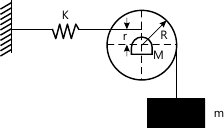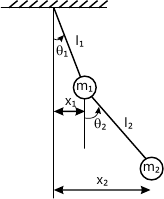MORE IN Dynamics of Machinery
SPPU Mechanical Engineering (Semester 7)
Dynamics of Machinery
December 2013
Total marks: --
Total time: --
INSTRUCTIONS
(1) Assume appropriate data and state your reasons
(2) Marks are given to the right of every question
(3) Draw neat diagrams wherever necessary

Answer any one question from Q1 and Q2
1 (a) Explain static and dynamic balancing and need of balancing.
6 M
1 (b) A three cylinder of an air compressor have their axes at 120° to each other and their connecting rods are coupled to a single crank. The stroke is 100 mm and length of each connecting rod is 150mm. The weight of reciprocating parts per cylinder is 15 N. Find the maximum primary and secondary forces acting on the frame of the compressor when running at 3000 RPM. Describe clearly a method by which such forces may be balanced.
10 M

2 A shaft is supported in bearings 1.8 m apart and projects 0.45 m beyond bearings at each end. The shaft carries three pulleys one at each end and one at the middle of its length. The mass of end pulleys is 48 kg and 20 kg and their centre of gravity are 15 mm and 12.5 mm respectively from the shaft axis. The centre pulley has a mass of 56 kg and its centre of gravity is 15 mm from the shaft axis. If the pulleys are arranged so as to give static balance, determine:
a) Relative angular positions of the pulleys, and
ii) Dynamic forces produced on the bearings when the shaft rotates at 300 r.p.m.
16 M

Answer any one question from Q3 and Q4
3 (a) Determine the natural frequency of oscillations of the simple pendulum considering the mass of rod.
8 M
3 (b) A mass of 1 kg is suspended by a spring passing over the pulley. The system is supported horizontally by spring of stiffness 1 kN/m. Determine the equation and natural frequency of vibration of a system. Using following data:
Mass of pulley M=10 kg;
Distance of spring from center of pulley r=35mm.10 M

4 (a) An under damped shock absorber is to be designed for a motorcycle of mass 200 kg such that during a road bump, the damped period of vibration is limited to 2 sec and amplitude of vibration should reduce to one-sixth in one cycle. Find spring stiffness and the damping coefficient of shock absorber.
10 M
4 (b) Define the following terms:
i) Logarithmic Decrement
ii) Damping coefficient
iii) Damping factor
iv) Coulomb damping
8 M

Answer any one question from Q5 and Q6
5 (a) Explain significance of Force transmissibility Versus Frequency ratio curve.
8 M
5 (b) A machine of 100kg is supported on spring of total stiffness 700 kN/m and has an unbalanced rotating element, which results in a disturbing force of 300 N at a speed of 2500 RPM. Assuming a damping factor of 0.25, determine
i) Amplitude of vibration
ii) Transmissibility
iii) Transmitted force
8 M

6 (a) A motor car moving with a speed of 100 kmph has a mass of 1500kg. It passes over a rough road which has a sinusoidal surface with amplitude of 75 mm and a wavelength of 5 m. The suspension system has spring constant of 500 N/mm and damping ratio of 0.5. Determine the displacement amplitude of the car.
8 M
6 (b) Explain the following terms.
i) Vibration Isolation
ii) Force Transmissibility
iii) Motion Transmissibility
iv) Magnification factor
8 M

Answer any one question from Q7 and Q8
7 (a) Set up the differential equations of motion for the double pendulum shown in following fig, using the coordinates x1 and x2 and assuming small amplitudes. Find the natural frequencies and ratios of amplitude, if m1=m2=m and 11=12.14 M
7 (b) Explain principal modes of vibration with respect to 2DOF translational system.
4 M

8 (a) Two rotors A and B are attached to the end of a shaft 500 mm long. Weight of the rotor A is 300 N and its radius of gyration is 300 mm. The corresponding values of rotor B are 500 N and 450 mm respectively. The shaft is 70 mm in diameter for the first 250 mm, 120 mm diameter for the next 100 mm and 100 mm diameter for the remainder of its length. Modulus of rigidity for the shaft material is 8×1011 N/m2. Find i) the position of the node and ii) the frequency of torsional vibration. Draw the mode shapes.
12 M
8 (b) Explain the concept of torsionally equivalent shaft and derive the relevant equation for it.
6 M

Answer any one question from Q9 and Q10
9 (a) Define the following terms:
i) Sound power level
ii) Sound pressure level
iii) Sound Intensity
iv) Decibel scale
4 M
9 (b) Explain human hearing mechanism with a neat sketch.
6 M
9 (c) A machinist working in a machine shop is operating 5 machines having their sound pressure levels as 95dB, 90dB, 92dB 88dB and 83dB, respectively. Determine the total sound pressure level when all 5 machines are turned on and when machine 4th & 5th are turned off.
6 M

10 (a) Explain the working of microphone.
4 M
10 (b) What is sound field ? What are the various types of sound fields in the vicinity of a sound source?
6 M
10 (c) What do you understand by sound enclosure? Describe the 2 types of sound enclosures
6 M

Answer any one question from Q11 and Q12
11 (a) What do you mean by vibration absorber? Explain the principle of operation of it.
4 M
11 (b) What do you mean by vibration isolation? What are the various methods of vibration isolation?
6 M
11 (c) A vibration measuring device is used to find the displacement, velocity and acceleration of a machine running at 120 RPM. If the natural frequency of the instrument is 5 Hz and it shows 0.04 mm. What are the 3 readings? Assume no damping.
6 M

Write short notes on the following:
12 (a) FFT Analyser
5 M
12 (b) Piezo electric accelerometer.
5 M
12 (c) Stroboscope
6 M

More question papers from Dynamics of Machinery